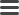# Model MatrixFeature Engineering tool

# Features Configuration

Model Matrix use Typesafe Config library for defining model matrix features and transformations.

Feature transformations documentations available in Model Matrix philosophy page.

## Extract expressions

Any valid Spark DataFrame (very limited subset of SQL) expression is supported as extract function for feature.

``````ad_performance = \${bins} {
...
extract = cast(ctr as double)
extract = ctr * 100
extract = nvl(ctr, 0)
...
}
``````

``````os = \${top} {
...
extract = concat('_', os_system, os_family)
...
}

...
...
}

...
...
}
``````

## Feature types

### Identity feature

``````identity_feature_name = {
active = <active status>
group = <feature group>
extract = <extract expression>
transform = "identity"
}
``````

### Top feature

``````top_feature_name = {
active = <active status>
group = <feature group>
extract = <extract expression>
transform = "top"
cover = <cover percentage>
allOther = <include all other>
}
``````

### Index feature

``````index_feature_name = {
active = <active status>
group = <feature group>
extract = <extract expression>
transform = "index"
support = <support percentage>
allOther = <include all other>
}
``````

### Binning feature

``````binning_feature_name = {
active = <active status>
group = <feature group>
extract = <extract expression>
transform = "bins"
nbins = <target number of bins>
}
``````

## Example Configuration

``````# Abstract Model Matrix features
feature = { active = true }

identity = \${feature} {
transform = "identity"
}

top = \${feature} {
transform = "top",
cover = 95.0
allOther = true
}

index = \${feature} {
transform = "index"
support = 0.5
allOther = true
}

bins = \${feature} {
transform = "bins"
nbins = 10
}

# Define concrete model matrix features
features {

extract = "network"
}

extract = "type"
}

extract = "size"
}